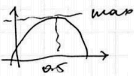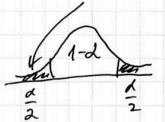# ML Wiki

## Binomial Proportion Confidence Intervals

These are Confidence Intervals for estimating a proportion in the population

• When we sample, we calculate a Point Estimate of the proportion
• We know that due to variance in the Sampling Distribution each time we get different estimates
• How we can expand the point estimate so it's likely to include the true value?

## Normal Approximation

This type of CI makes use of Central Limit Theorem and Normal Approximation of Binomial Distribution

So, for any experiment, let

• $p$ be the true probability
• $n$ be the number of trials

Then

• estimate $p$ as $\hat{p} = \cfrac{\text{success}}{n}$
• and the CI is $\left[\hat{p} - 1.96 \sqrt{p(1-p)/n}; \hat{p} + 1.96 \sqrt{p(1-p)/n}\right]$

### Building a Confidence Interval

• We have a sample of $n$ observations: $X_1, ..., X_{n}$
• let $\hat{p} =$ fraction of successful $X_i$, i.e. $\hat{p} = \cfrac{\text{# of success}}{n}$
• so we calculate $\hat{p}$ based on the data in the sample
• if all observations are independent and probability of success $p$ is always the same, then Sampling Distribution is Binomial Distribution
• i.e. each $X_i \sim \text{Bernoulli}(p)$, variance $\text{Var}[X_i] = p \cdot (1 - p) = pq$

Parameters of the Sampling Distribution

• $\hat{p}$ is an Unbiased Estimate of $p$: $E[\hat{p}] = p$
• $E[X_i] = p, \hat{p} = \cfrac{1}{n} \sum_{i=1}^n X_i$
• $E[\hat{p}] = E \left[ \cfrac{1}{n} \sum_{i=1}^n X_i \right] = \cfrac{1}{n} \sum_{i=1}^n E [ X_i ] = \cfrac{np}{n} = p$
• $\text{var}[\hat{p}] = \cfrac{p(1-p)}{n}$
• $\text{var}[\hat{p}] = \text{var} \left[ \cfrac{1}{n} \sum_{i=1}^n X_i \right] = \cfrac{1}{n^2} \sum_{i=1}^n \text{var}[X_i] = \cfrac{npq}{n^2} = \cfrac{pq}{n} = \cfrac{p(1-p)}{n}$
• $\text{sd}[ \hat{p} ] = \sqrt{ \cfrac{p \cdot (1 - p)}{n} }$
• Now we use the Normal Approximation (i.e. apply the C.L.T. and calculate that the SD follows Normal Distribution $N \left( \mu=p, \sigma = \sqrt{ \cfrac{p(1-p)}{n} } \right)$)

We want to build CI at level of $\alpha$

E.g. 95% CI

• $z = 1.96$ and we know that 95% of the values lie in $(-z, +z)$
•• So only in 5 experiments out of 100 you end up outside of this interval

R code to produce the figure
x = seq(-3, 3, 0.1)
y = dnorm(x)

plot(x, y, type='l', bty='n', main='95% CI on N(0,1)')

x1 = min(which(x > -1.96)); x2 = max(which(x < 1.96))
polygon(x[c(x1, x1:x2, x2)],

text(x=0, y=0.2, labels='0.95', cex=1.5)
text(x=c(-2.07, 2.07), y=0.025, labels='0.025', cex=0.6)


This is called 95% confidence interval for $p$:

• $\left[\hat{p} - 1.96 \sqrt{p(1-p)/n}; \hat{p} + 1.96 \sqrt{p(1-p)/n}\right]$
• left part - lower bound
• right part - upper bound

We say that we're 95% confident that the true value of $p$ is somewhere in this interval.

### Margin of Error

Problem: $p$ (to use under the square root) is unknown!

Solutions:

• use $\hat{p}$ instead of $p$ (we assume it should be close) or
• use $p = q = 0.5$: it maximizes our margin of error
•• margin of error is $\beta = 1.96 \sqrt{p(1-p)/n}$

### Critical Value

Why we chose 95% CI with $\alpha = 0.05$ and not another one?

We can compute any confidence interval using any $\alpha$

• Compute critical value $z_{\alpha/2}$ such that "not interesting" areas under the normal curve take $\alpha / 2$
•• so the interval will be $\left[-z_{\alpha/2}; z_{\alpha/2}\right]$ and the "interesting" area under the bell curve is $1 - \alpha$

Margin of error is this case is

• $\beta = z_{\alpha/2} \sqrt{p(1-p)/n}$ and, as we know, for $95\%$, $\alpha = 0.025$ and $z_{0.025} = 1.96$

As we see, the CI becomes wider as critical value grows

### Assumptions

Note that by using the C.L.T. we assume that:

he central limit theorem applies poorly to this distribution with a sample size less than 30 or where the proportion is close to 0 or 1. The normal approximation fails totally when the sample proportion is exactly zero or exactly one. A frequently cited rule of thumb is that the normal approximation is a reasonable one as long as np > 5 and n(1 − p) > 5;

## Examples

### Flipping a Beer Cap

Imagine an experiment where we flip a beer cap

• it follows the Binomial Distribution, but we don't know the true parameter $p$
• say we flipped a beer cap 1000 times and got 576 reds: $\hat{p} = 0.576$
• what is its statistical model? What is $p$ in $\text{Binomial}(1000, p)$?

Let's build a Confidence Interval for that

• so we estimate $\hat{p} = 0.576$ and $\text{Var}(\hat{p}) = \cfrac{p(1-p)}{n} = \cfrac{p(1-p)}{1000}$

Result:

• 95% CI is $[0.545, 0.607]$

In R:

phat = 0.576
z = qnorm(0.025, mean=0, sd=1, lower.tail=F) // the right tail rather then left
ME = z * sqrt(phat * (1- phat) / 1000) // Margin of error: we replace p by phat
CI = phat + c(-ME, ME) // 0.545, 0.606


### Example 2

• Calculate the 90% CI for $p$
• With 60 successes out of 100 trials
phat = 0.6
cl = 0.9
al = (1 - cl) / 2
z = qnorm(al, mean=0, sd=1, lower.tail=F)
n = 100

ME = 2 * sqrt(0.5 * 0.5 / n)
ci = phat + c(-ME, ME)
// [0.52, 0.68]